Example Questions

A physicist wishes to study the trajectory of a ball launched horizontally. She varies parameters such as the launching velocity, starting height, and mass of the ball. For each trajectory, she records the time of flight (in seconds) and horizontal displacement (in meters). She assumes air resistance is negligible.

Figure 1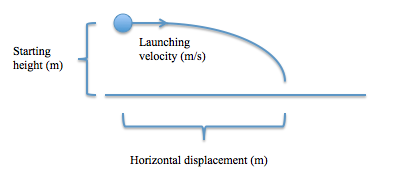Using all of the data she collects, she constructs the following table:

Table 1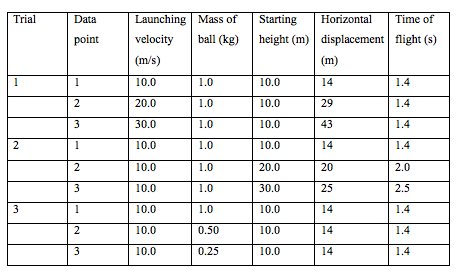Which of the following variables were controlled in Trial 2?

Mass of the ball and launching velocity

Starting height and launching velocity

Mass of the ball only

Starting height only

Mass of the ball and launching velocity

Explanation:

In Trial 2, only the starting height varies, as the mass of the ball and launching velocity remain constant.

A particle separator functions by using a magnetic field and an electric field pointing perpendicularly. When a charged particle is launched into the particle separator, a constant electric force is exerted on the particle proportional to the particle's charge. Additionally, the magnetic field exerts a force on the particle that is in the opposite direction of the electric force and that is proportional to the particle's charge and velocity. A particle will make it through the particle separator only if the opposing magnetic forces and electric forces are equal in magnitude as they will not cause a net change in the particle's direction.

According to the information of the passage, by what physical characteristic does a particle separator separate particles?

Mass

Velocity, charge, and mass

Velocity and charge

Velocity

Charge

Velocity

Explanation:

The correct answer is velocity. This is a difficult question because it requires critical consideration of the passage information. In the passage, we learn that both the magnetic and electric forces are proportional to the particle's charge. However, we also learn that only the magnetic force is proportional to the particle's velocity. Therefore, we know that no matter what charge a particle has, it will make it through the particle separator only if it has the right velocity such that the magnetic force produced is enough to counter the electric force on the particle. Mass is not mentioned in the passage, so we can ignore any answer including mass.

A particle separator functions by using a magnetic field and an electric field pointing perpendicularly. When a charged particle is launched into the particle separator, a constant electric force is exerted on the particle proportional to the particle's charge. Additionally, the magnetic field exerts a force on the particle that is in the opposite direction of the electric force and that is proportional to the particle's charge and velocity. A particle will make it through the particle separator only if the opposing magnetic forces and electric forces are equal in magnitude as they will not cause a net change in the particle's direction.

What can we infer would happen if a neutron (a particle with no charge) were to be shot into the particle separator with a low velocity?

It would be repelled from the particle separator in the opposite direction from which it was launched.

It would move towards the direction of the magnetic force.

It would move towards the direction of the electric force.

It would make it through the particle separator.

It would quickly slow to a stop.

It would make it through the particle separator.

Explanation:

The correct answer is that it would make it through the separator. As stated in the passage, the force of the magnetic and electric fields on the particle are proportional to the charge of the particle. Therefore, a neutral particle (with charge = 0) would produce no force from either field. Therefore, the velocity of the particle would remain the same and it would (slowly) make it out of the separator without changing its direction.

A particle separator functions by using a magnetic field and an electric field pointing perpendicularly. When a charged particle is launched into the particle separator, a constant electric force is exerted on the particle proportional to the particle's charge. Additionally, the magnetic field exerts a force on the particle that is in the opposite direction of the electric force and that is proportional to the particle's charge and velocity. A particle will make it through the particle separator only if the opposing magnetic forces and electric forces are equal in magnitude as they will not cause a net change in the particle's direction.

Which of the following statements about the electric field and magnetic field strength is consistent with the information provided in the passage?

For the particle separator to function, the electric field must always be stronger than the magnetic field.

For the particle separator to function, the ratio of the strength of the electric field to the strength of the magnetic field must vary randomly.

For the particle separator to function, the ratio of the strength of the electric field to the strength of the magnetic field must remain constant.

For the particle separator to function, the ratio of the strength of the electric field to the strength of the magnetic field must vary with time.

For the particle separator to function, the magnetic field must always be stronger than the electric field.

For the particle separator to function, the ratio of the strength of the electric field to the strength of the magnetic field must remain constant.

Explanation:

This question requires reading the passage closely. The passage reveals that the particle separator separates particles according only to their velocity. The way this is accomplished is that while particles are flowing through the separator, particles that are going faster (have a greater velocity) experience a greater magnetic force opposite of the electric force and therefore only having a specific velocity (with which a particle experiences equal magnetic and electric forces) will allow a particle to exit the separator and not be pushed in one direction or the other. No matter what the ratio of the magnetic field to the electric field is, it must remain constant, or else the forces on each particle will vary according to the particles' velocities and the changing magnetic and electric fields. Therefore, in order for this apparatus to function correctly, a constant magnetic to electric ratio is necessary.

An engineer wants to design a prank toy that will be thrown onto the ground. It looks like a superball (bouncing ball), but does not bounce. The engineer has Subtance A, Substance B, and Substance C that he drops from various heights. All of the tests are performed by dropping the substances on the same surface and each height is tested multiple times. The error between experiments is minimal and too small to be seen on the graph. The engineer records the maximum rebound height of each substance at the various drop heights. This data is displayed in the graph below.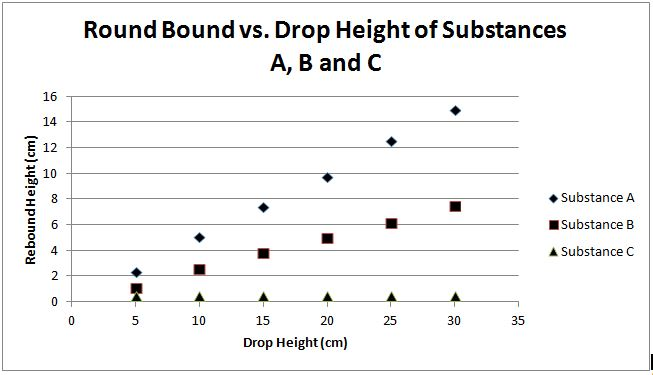Which of the following will be the best improvement that can be made in the design of the experiment?

Perform the experiment by throwing the substance onto the surface instead of just dropping the substance

All of the choices would be great improvements on the experiment

Test the substance at more drop heights within the tested range

Record the time it takes each substance to reach its peak rebound height

Test more substances

Perform the experiment by throwing the substance onto the surface instead of just dropping the substance

Explanation:

Substance C appears to achieve the desired goal of a small rebound height, which eliminates the need to test more substances. Recording the time that it takes each substance to reach it maximum rebound height does not add any necessary information to the experiment. This fact eliminates that option. A linear relationship can be seen from the already tested data points and there is no need to perform more experiments at different drop heights from the initial experiment. This also eliminates the option for all of the choices being improvements on the experiment. This leaves the correct answer of throwing the substances instead of just dropping them.

Alternatively, the substances are used to be prank superballs that will thrown onto the ground. The substances that will be used for making the prank superball will not simply be dropped, it will be thrown into the ground. Throwing the substances will give more accurate experimental data by taking into account this added force not present in the drop experiment.

Engineers are evaluating four potential technologies. These technologies are to be used as power plants that are considered "clean" energy. The estimated energy output of these plants were calculated and the resources needed to run these were also listed.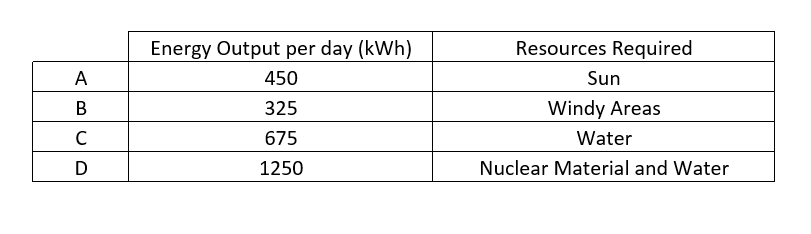Which of the following should the engineers also research before building the power plant?

Customer views towards each technology

All of these

Safety of each technology

The cost of building and resource require for the facility for each option

All of these

Explanation:

The engineers would need to know the funds/budget that they have at their disposal to see which technology they can pursue. The engineers would then need to calculate the cost of building and running such a facility. This would dictate if the engineers were able to actually build and use the facility without raising the cost too much to customers. Finally, some customers in certain areas may strongly oppose certain types of technology in their neighborhood. From this logic the engineers should consider customers' opinions. The safety of each technology is necessary to not endanger the lives of the workers at each plant. Since all of these are important to know the engineers should research all of these topics.

A company developed new prototype car and wanted to test the new car. The car's acceleration time from 0mph to 60mph and stopping time from 60mph to 0mph were measured. An obstacle course involving a lot of turns was also constructed to determine how well the car can handle turning. The main concern of the company is the safety of the car and therefore all of the tests were performed on dry concrete, concrete after simulated rain, concrete that was covered with snow and in sand.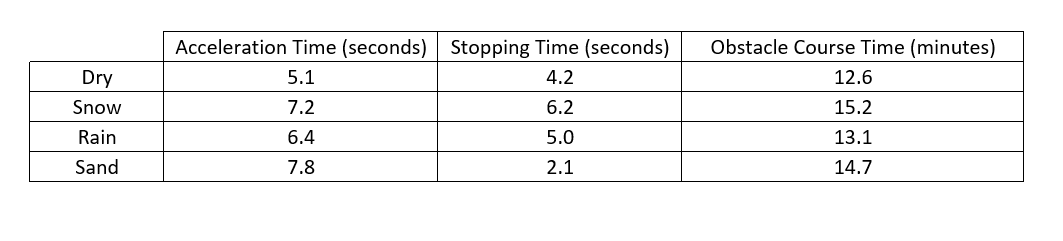What other aspects of the car should this company test?

Determine the miles per gallon that the car gets

Test the top speed of the car

Determine if consumers like the style of the car

Obtain a safety rating for the car

Obtain a safety rating for the car

Explanation:

It is stated that the main concern of the car company is the safety of the car. Therefore the car company should obtain a safety rating and make sure that the safety of the car is up to necessary standards. Determining the miles per a gallon, top speed and the ideal style of the car would be helpful to the company, but will not help determine if the car is safe for consumers. The correct answer is obtain a safety rating for the car.

Two scientists are concerned with producing electricity with magnets.

Scientist 1 believes that rotating a magnet within a coil of wires will produce electricity. Scientist 1 asserts that the larger the magnet the more electricity will be produced.

Scientist 2 believes that rotating the coil of wires around a magnet will produce electricity. Scientist 2 asserts that the more number of coils the more electricity will be produced.

The scientists conduct the following experiment. The scientists take four magnets, A, B, C and D; with A being the smallest magnet, B the next smallest, then C and the largest magnet was D. The scientist rotate the magnets at the same constant speed in the coils and record the number of milliamps produced. This was done in copper wiring with 20, 40 and 60 coils. When testing by rotating the coil around the magnet at the same speed of the magnet rotation produced the results recorded below.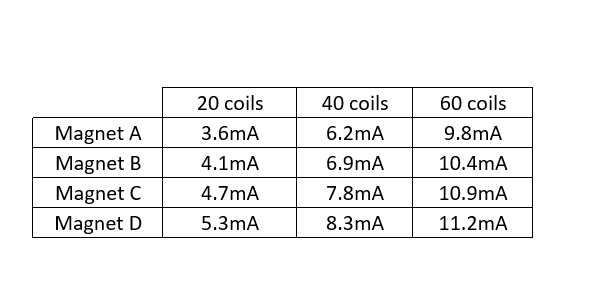What else can be tested to be determine ways to increase the maximum current produced?

All of these

The rotation speed of the coil or magnet

The material of the coil

The distance between the coil and the wiring during rotation

All of these

Explanation:

The rotation speed of the coil or magnet could affect the output of the current, but there is no information on this. This should be tested. The scientist never state what material the coil was made of and this compared to other conducting materials could impact the current produced. In the experiments it is unclear how the spacing between the magnets and the coils affect the results and should also be tested.

A student is performing a science experiment for his class. The student creates a ramp that contains four different surfaces, carpet, glass, wood and plastic. The ramp is held at a constant angle and a ball is allowed to roll down the ramp. The student tests each material by releasing a ball at different distances from the bottom of the ramp and recording the time it takes for the ball to travel the distance of the ramp.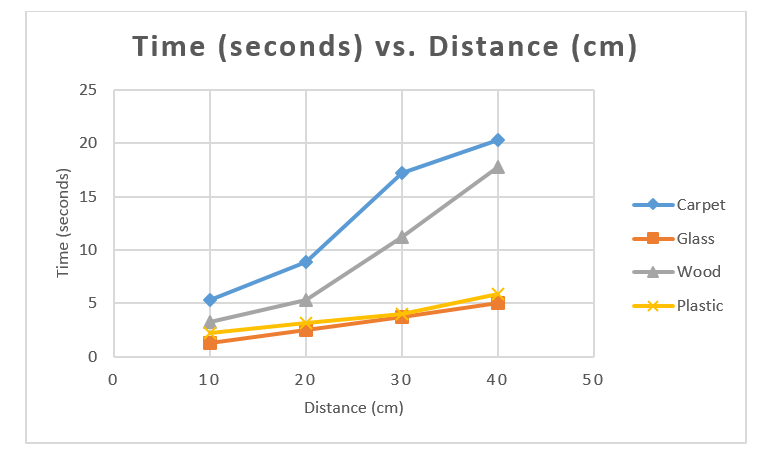What is/are the independent variable(s) in this experiment?

The time it takes the ball to travel down the ramp

The material of the surface and the time it takes the ball to travel down the ramp from the release height

There are no independent variables

The material surface and the height at which the ball is released

The material surface and the height at which the ball is released

Explanation:

An independent variable is something that is not affect by the experiment. In other words the independent variables are not affected by the other variables in the experiment. The time it takes for the ball to travel down the ramp is affected by the distance and the material of the surface. Therefore the time variable is the dependent variable. The distance the ball travels and the material surface tested are not affected by any other variables and are the independent variables.

Sometimes scientists need to scale down experiments because collecting full-scale data may not be practical or possible. Most of the time, the data collected during a small scale-experiment is an accurate indicator of full-scale results. For example, research of craters on the Earth’s surface has indicated that the Earth has experienced a history of massive meteor collisions. It has been hypothesized that, amongst other things, these impacting meteorites caused the Earth’s oceans to vaporize. The detrimental effects associated with meteor collisions would have made the planet to be uninhabitable to humans. Due to these adverse effects, an experiment that could produce meteor-sized craters would not be possible in a full-scale setting.

Researchers have developed small-scale experiments that are exemplary of Newton’s three laws of motion. Newton’s first law states that an object in motion will stay in motion unless it is acted on by another force. This phenomenon is known as inertia. The second law comes in the form of an equation that calculates how much force is exerted on two colliding objects with respect to mass and acceleration. In other words force is equal to the mass of an object multiplied by its acceleration. The third law states that for every action there is an equal and opposite reaction. In other words, forces always occur in pairs. A counter force that is equal in magnitude and opposite in direction compliments each force in a particular direction. For example, if you push downwards on a table then the table, in theory, pushes upwards with the same force.

In a particular small-scale exercise, students were asked to design an experiment in which two “meteorites” would be dropped into a medium of sand and the subsequent crater diameters would be measured. Afterwards, the sand would be leveled and the experiment would be repeated. The students were asked to identify all of the factors that could affect the formation of the craters. This was done in order to ensure that each experiment was testing one independent and one dependent variable at a time. Otherwise, it would be unclear which variable is causing the observed effect. Possible variables that could alter the outcome of the experiment included but were not limited to the following: height of the fall, speed of the “meteorite,” angle of impact, and the mass of meteorite.

Consider an experiment that seeks to identify the mass of the meteorite analog. All other variables would have to remain constant in order to ensure accurate results.  In order to do this, the height of the fall was set to one meter. The object was placed on top of a vertical meter stick. In order to keep the acceleration and height of the drop constant, it was rolled off of the stick instead of being pushed or simply dropped. Last, a protractor was used to keep the meter stick at a ninety-degree angle. In the procedure, a marble and a golf ball were used as “meteorites” of different masses.

The results of the experiment were recorded in the table and figure provided.

 Crater Diameter (cm) Trial Number Marble (mass = 4g) Golf ball (mass = 46g) 1 4.5 7.75 2 4.5 6 3 4 5.5 4 3 6 5 3 6.5 6 3.5 7 7 3 5 8 3 5 9 3 7 10 3.5 6 Average Average 3.5 cm 6.175 cm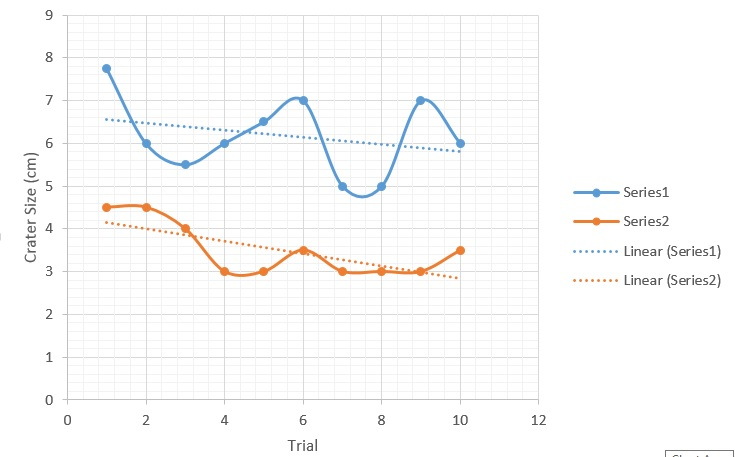Which of the following choices most accurately identifies the independent and dependent variables of this experiment?

Independent Variable: Velocity

Dependent Variable: Height

Independent Variable: Crater size

Dependent Variable: Mass

Independent Variable: Mass

Dependent Variable: Velocity

Independent Variable: Mass

Dependent Variable: Crater size